## How to Convert Uppercase to Lowercase Except the First Letter in Excel

In excel, words can be entered in uppercase, lowercase or mixed. If we want to convert all uppercase to lowercase (except the first letter in some situations), we can use formula with some letter convert related functions to convert them to proper case. This article will introduce you some methods to convert letters from uppercase to lowercase, it can help you to solve your problem.

Precondition:

Prepare below table. To convert uppercase to lowercase for worlds or sentence, actually there are two forms after converting. We can convert uppercase to lowercase but keep the first uppercase for each word; in another way, we can convert uppercase to lowercase but only keep the first uppercase for the first word in the sentence.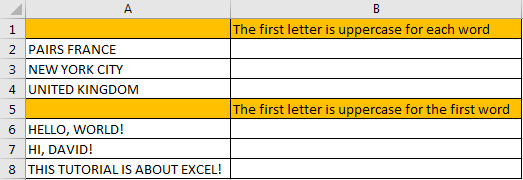## Part 1: Convert Uppercase to Lowercase Except the First Letter for Each Word

Step 1: In B2 enter the formula =PROPER(A2).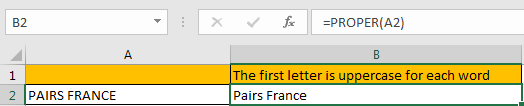For PROPER function, it converts a text or string to proper case, actually it converts the first letter in each word to uppercase, but keeps other letters in lowercase. So, no matter letters are entered in uppercase or lowercase or mixed, it will be finally displayed with the first letter in uppercase with the other letters in lowercase after applying this function.

Step 2: Drag the fill handle down to fill other cells. Verify that uppercase is converted to lowercase properly.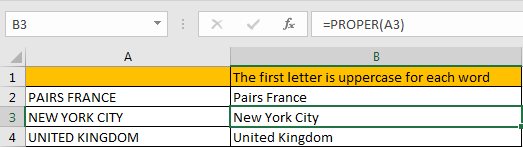## Part 2: Convert Uppercase to Lowercase Except the First Letter for the First Word

Step 1: In B6 enter the formula =UPPER(LEFT(A6,1))&LOWER(RIGHT(A6,LEN(A6)-1)).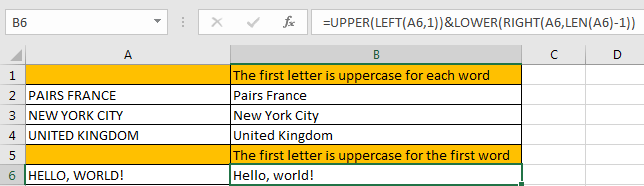This formula is combined with UPPER and LOWER two functions. It is easy to understand. For UPPER function, keep the first letter from left displaying in uppercase; for LOWER function, keep other letters except the first letter from left displaying in lowercase.

Step 2: Drag the fill handle down to fill other cells. Verify that uppercase is converted to lowercase properly.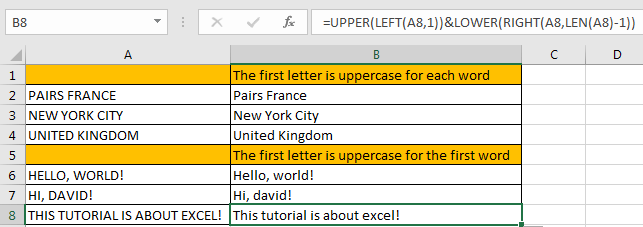### Related Functions

• Excel UPPER function
The Excel UPPER function converts all characters in text string to uppercase.The UPPER function is a build-in function in Microsoft Excel and it is categorized as a Text Function.The syntax of the UPPER function is as below:= UPPER (text)…
• Excel LOWER function
The Excel LOWER function converts all characters in a text string to lowercase.The syntax of the LOWER function is as below:= LOWER  (text)…
• Excel LEFT function
The Excel LEFT function returns a substring (a specified number of the characters) from a text string, starting from the leftmost character.The LEFT function is a build-in function in Microsoft Excel and it is categorized as a Text Function.The syntax of the LEFT function is as below:= LEFT(text,[num_chars])…
• Excel RIGHT function
The Excel RIGHT function returns a substring (a specified number of the characters) from a text string, starting from the rightmost character.The syntax of the RIGHT function is as below:= RIGHT (text,[num_chars])…
• Excel LEN function
The Excel LEN function returns the length of a text string (the number of characters in a text string).The syntax of the LEN function is as below:= LEN(text)…

## How To Force Text String to Upper,Lower, or Proper Case in Excel

This post will guide you how to force test strings to uppercase or lowercase or Proper cases through an Excel formula or data validation in your Excel 2013/2016. How to convert text to upper, lower, or proper case in Excel.

Assuming that you want to force text strings to Upper case when you type words into one cell, and how to do it. You can use an Excel function in Data Validation feature to achieve it. Just do the following steps:

Step1: go to DATA tab, click Data Validation command under the Data Tools group. Then clicking on the Data Validation menu from the drop down menu list. Then the Data Validation window will appear.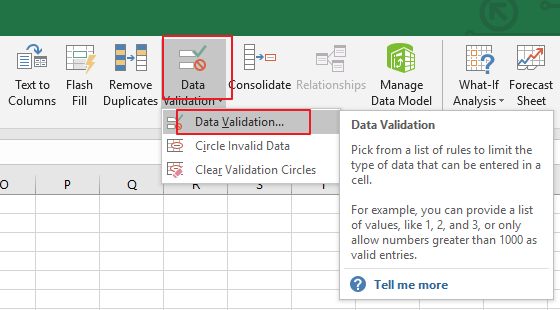Step2: in the Data Validation dialog box, and switch to Settings tab, and click the Allow drop down list and choose Custom value from the drop down menu list.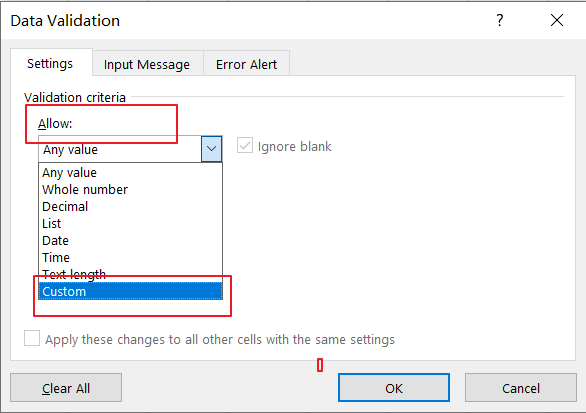Step3: Then type the following formula in the Formula text box in the Data Validation dialog box.

Note:

If you want to convert text string to Upper Case, type the following formula:

=EXACT(UPPER(B1),B1)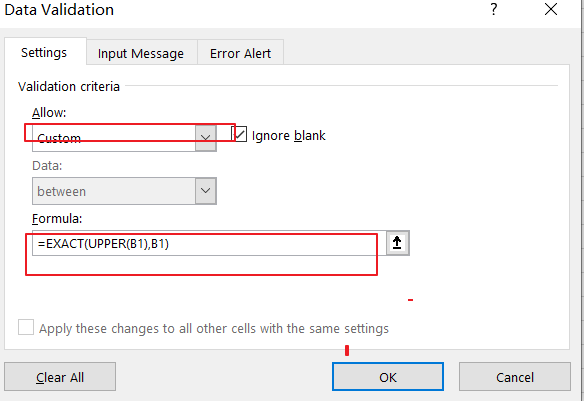If you want to convert text string to Lower Case, just use the following formula:

=EXACT(LOWER(B1),B1)

If you want to convert text string to Proper Case, just use the following formula:

=EXACT(PROPER(B1),B1)

Step4: then you need to switch to Error Alert tab in the Data Validation dialog box. And select Stop value from the Style drop down list box, and type some error message under Error message text box. Then click on Ok button.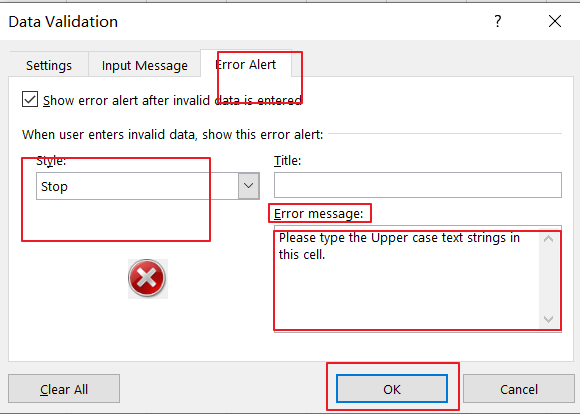Step5: you can try to enter one text string in cell B1 to verify if it take effect.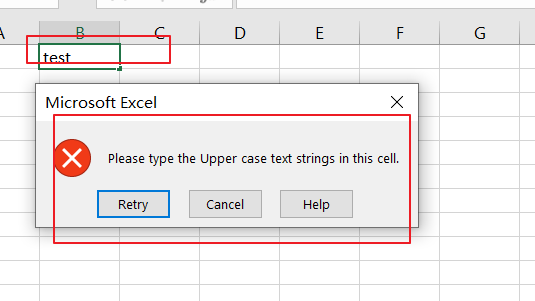You can also use an Excel VBA Macro to achieve the same result of forcing Text string to Upper, Lower or Proper cases in you worksheet. Just do the following steps:

Step1: open your excel workbook and then click on “`Visual Basic`” command under `DEVELOPER` Tab, or just press “`ALT+F11`” shortcut.Step2: then the “`Visual Basic Editor`” window will appear.

Step3: choose on your current worksheet from the left Project Explorer, and double click on it to open the code window, and the copy and paste the following VBA code into it.

For Upper Case:

```Private Sub Worksheet_Change(ByVal Target As Range)

Target.Value = VBA.UCase(Target.Value)

End Sub```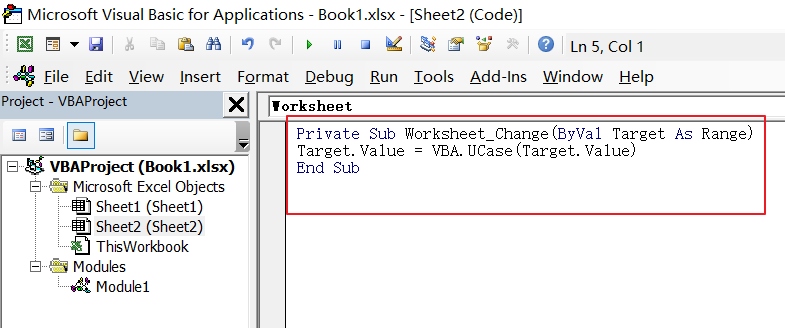For Lower Case:

```Private Sub Worksheet_Change(ByVal Target As Range)

Target.Value = VBA. LCase (Target.Value)

End Sub```

For Proper Case:

```Private Sub Worksheet_Change(ByVal Target As Range)

Target.Value = Application.WorksheetFunction.Proper(Target.Value)

End Sub```

Step4: save and close the current VBA window. And then you can try to enter one text string to verify it. when you type one word when it is lower or proper cases, and it will convert it to Uppercase.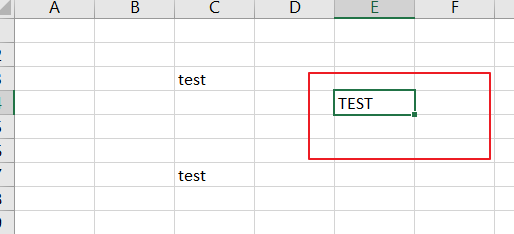### Related Functions

• Excel UPPER function
The Excel UPPER function converts all characters in text string to uppercase.The UPPER function is a build-in function in Microsoft Excel and it is categorized as a Text Function.The syntax of the UPPER function is as below:= UPPER (text)…
• Excel LOWER function
The Excel LOWER function converts all characters in a text string to lowercase.The syntax of the LOWER function is as below:= LOWER  (text)…
• Excel Proper Function
The Excel PROPER function capitalizes the first character in each word of a text string and set other characters to lowercase. The syntax of the PROPER function is as below:= PROPER  (text)…
• Excel EXACT function
The Excel EXACT function compares if two text strings are the same and returns TRUE if they are the same, Or, it will return FALSE.The syntax of the EXACT function is as below:= EXACT (text1,text2)…

## How to Remove Middle Name from Full Name in Excel

When we get a list of full names, we can remove the middle name for short in some cases. As the middle names are different among the full name list so we cannot replace them by space directly in excel. This article will provide you two methods to remove the middle name. User can use Formula to remove the middle name, or can remove it by Find and Replace function.

Prepare a list of full names.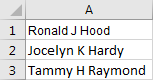## Remove the Middle Name from Full Name by Formula

Step1: Select a blank cell next to the full name, for example select B1. Enter the following formula into B1:

=TRIM(LEFT(A1,FIND(” “,LOWER(A1),1))) & ” ” & TRIM(MID(A1,FIND(” “,LOWER(A1),FIND(” “,LOWER(A1),1)+1)+1,LEN(A1)-FIND(” “,LOWER(A1),1)+1)) .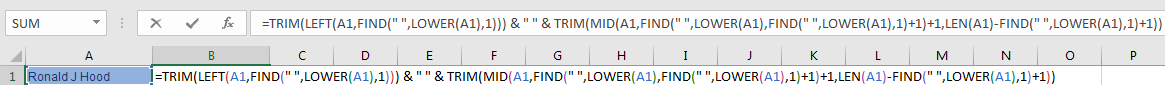Step2: Click Enter and get the result.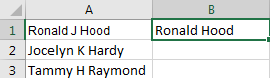Step3: Drag the Auto Fill handle to fill B2 and B3. Now all the middle names are removed from the list.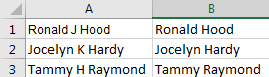## Remove the Middle Name from Full Name by Find and Replace Function

Step1: Click Ctrl+F to load Find and Replace window.

Step2: Under Replace tab, in Find what textbox enter ‘ * ‘ (a space + * + a space); in Replace with textbox enter ‘ ‘ (a space). Then click Replace All.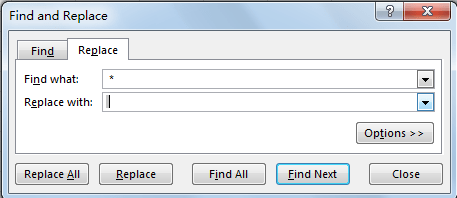Step3: Check the result. All the middle names are removed.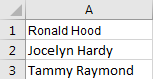### Related Functions

• Excel LEFT function
The Excel LEFT function returns a substring (a specified number of the characters) from a text string, starting from the leftmost character.The LEFT function is a build-in function in Microsoft Excel and it is categorized as a Text Function.The syntax of the LEFT function is as below:= LEFT(text,[num_chars])…t)…
• Excel FIND function
The Excel FIND function returns the position of the first text string (sub string) within another text string.The syntax of the FIND function is as below:= FIND(find_text, within_text,[start_num])…
• Excel TRIM function
The Excel TRIM function removes all spaces from text string except for single spaces between words.  You can use the TRIM function to remove extra spaces between words in a string.The syntax of the TRIM function is as below:= TRIM (text)….
• Excel LEN function
The Excel LEN function returns the length of a text string (the number of characters in a text string).The syntax of the LEN function is as below:= LEN(text)…
• Excel LOWER function
The Excel LOWER function converts all characters in a text string to lowercase.The syntax of the LOWER function is as below:= LOWER  (text)…

## How to Check If a Cell is Uppercase, Lowercases or Capitalize in Excel

This post will guide you how to check if a cell is uppercase in Excel 2013/2016. How do I identify all letters in cells are lowercases or capitalize in Excel. How to change letters to uppercase, lowercases or capitalize first letter for all words in cells in Excel.

## Check If All Letters are Uppercase

If you want to check if all letters in cells are uppercase, you can use a formula based on the EXACT function and the UPPER function. Like this:

`=EXACT(B1,UPPER(B1))`

Type this formula into a blank cell and press Enter key to apply this formula. If All letters are uppercases, and it returns TRUE. Otherwise, it returns FALSE. Then drag the AutoFill Handle down to other cells to apply this formula.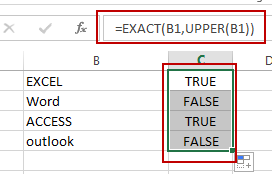Let’ see how this formula works:

The UPPER function will convert all letters to uppercase. And then pass the result to EXACT function as its second argument. And the EXACT function will compare if two values are equal. If equal, returns TRUE. Otherwise, returns FALSE.

## Check If All Letters are Lowercase

If you need to identify all characters in cells are lowercase, you can use a formula based on the LOWER function and the EXACT function. Like this:

`=EXACT(B1,LOWER(B1))`

Type this formula into cell C1, and press Enter key to apply this formula. If all letters are lowercases, and it returns TRUE. Otherwise, it returns FALSE. Then drag the AutoFill Handle down to other cells to apply this formula.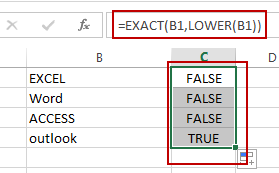The LOWER function converts all letters to lowercase.

## Check If the First Letters are capitalized

If you want to check if the first character in each word is capitalized, you can use a formula based on the EXACT function and the PROPER function. Like this:

`=EXACT(B1,PROPER(B1))`

Type this formula into cell C1, and press Enter key to apply this formula. If all the first letters are capitalized, and it returns TRUE. Otherwise, it returns FALSE. Then drag the AutoFill Handle down to other cells to apply this formula.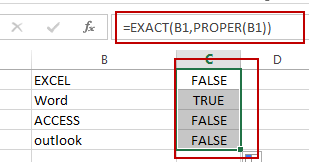The PROPER function will capitalize the first letter in each word in Cell.

### Related Functions

• Excel UPPER function
The Excel UPPER function converts all characters in text string to uppercase.The UPPER function is a build-in function in Microsoft Excel and it is categorized as a Text Function.The syntax of the UPPER function is as below:= UPPER (text)…
• Excel LOWER function
The Excel LOWER function converts all characters in a text string to lowercase.The syntax of the LOWER function is as below:= LOWER  (text)…
• Excel Proper Function
The Excel PROPER function capitalizes the first character in each word of a text string and set other characters to lowercase. The syntax of the PROPER function is as below:= PROPER  (text)…
• Excel EXACT function
The Excel EXACT function compares if two text strings are the same and returns TRUE if they are the same, Or, it will return FALSE.The syntax of the EXACT function is as below:= EXACT (text1,text2)…

## Change Uppercase and Lowercase Text

This post will guide you how to change the case of text to uppercase or lowercase in Excel. How do I change all uppercase text to lower case with an Excel formula.

For example, if you want to change all text string in uppercase into lowercase, you can create a formula based on the Lower function. And if you want to convert all letters in one cell into uppercase, you can use the Upper function as a formula in excel.

If you want to show in Cell C1 a lowercase version of the text in Cell B1, you can type the following formula into the formula box in Cell C1.

=LOWER(B1)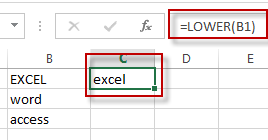If you want to show in Cell C2 an uppercase version of the text in B2, you could type the below formula into the formula box in C2.

=UPPER(B2)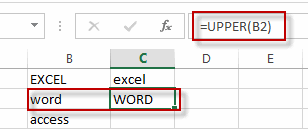If you want to show in Cell C3 a proper version of the text in B3, you could type the below formula into the formula box in C3:

=PROPER(B3)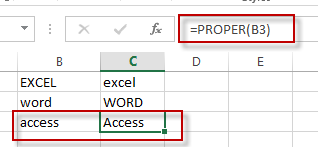### Related Functions

• Excel UPPER function
The Excel UPPER function converts all characters in text string to uppercase.The UPPER function is a build-in function in Microsoft Excel and it is categorized as a Text Function.The syntax of the UPPER function is as below:= UPPER (text)…
• Excel LOWER function
The Excel LOWER function converts all characters in a text string to lowercase.The syntax of the LOWER function is as below:= LOWER  (text)…
• Excel Proper Function
The Excel PROPER function capitalizes the first character in each word of a text string and set other characters to lowercase. The syntax of the PROPER function is as below:= PROPER  (text)…

## Excel Lower Function

This post will guide you how to use Excel LOWER function with syntax and examples in Microsoft excel.

### Description

The Excel LOWER function converts all characters in a text string to lowercase.

The LOWER function is a build-in function in Microsoft Excel and it is categorized as a Text Function.

The LOWER function is available in Excel 2016, Excel 2013, Excel 2010, Excel 2007, Excel 2003, Excel XP, Excel 2000, Excel 2011 for Mac.

### Syntax

The syntax of the LOWER function is as below:

= LOWER  (text)

Where the LOWER function arguments are:
text -This is a required argument. The text string that you want to convert to lowercase.

### Example

The below examples will show you how to use Excel LOWER Text function to convert all uppercase characters in a text string to lowercase.

#1 To lower case all characters in B1 cell, just using the following formula:=LOWER(B1).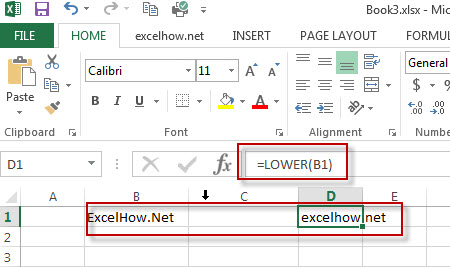### More Related LOWER Function Examples

• Change Uppercase and Lowercase Text
if you want to change all text string in uppercase into lowercase, you can create a formula based on the Lower function. And if you want to convert all letters in one cell into uppercase, you can use the Upper function as a formula in excel.…